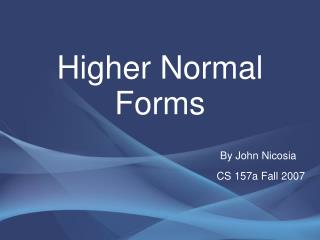DownloadDownload PresentationHigher Normal Forms

Higher Normal Forms

Télécharger la présentationHigher Normal Forms

- - - - - - - - - - - - - - - - - - - - - - - - - - - E N D - - - - - - - - - - - - - - - - - - - - - - - - - - -
Presentation Transcript

1. Higher Normal Forms By John Nicosia CS 157a Fall 2007

2. What I Will Talk About Define Multi-valued Dependency (MVD) MVD v. FD Armstrong's Axioms for MVD Quick Review of 1NF, 2NF, 3NF, BCNF Fourth Normal Form (4NF)

3. Multi-valued Dependency (MVD) The multivalued dependency X --> --> Y holds in a relation R if whenever we have two tuples of R that agree in all the attributes of X, then we can swap their Y components and get two new tuples that are also in R. Trivial: If Y is a subset of X or X U Y is all the attributes of R. Non-Trivial: If Y is not a subset of X and X U Y is not all the attributes of R.

4. MVD v. FD

5. MVD v. FD (cont) Functional Dependency: 1. Every FD is a MVD. 2. FDs rule out certain tuples * If X --> Y then we can't have two tuples with the same X values but different Y values.

6. MVD v. FD (cont) Multi-Valued Dependency: 1. Not every MVD is a FD. 2. MVDs guarantee certain tuples exist. *If Name--> --> PhoneNum

7. How To Test for MVD

8. How To Test for MVD (cont)

9. How To Test for MVD (cont)

10. How To Test for MVD (cont)

11. How To Test for MVD (cont)

12. Armstrong's Axioms for MVD Reflexivity Rule: if , then A -->-->B Complementation Rule: if A-->-->B, then A -->--> [R - ( )] Augmentation Rule: if A-->--> B and then (A,D) -->--> (B,C) Transitivity Rule: if A-->-->B and B-->-->C then A-->-->(C-B) Replication Rule: if A-->B then A-->--->B Coalescence Rule: if A-->-->B, (B, D) are disjoint, D--> C, then A-->C

13. Quick Review of Normal Forms 1NF: All values of the attributes contain no repeating values. 2NF: 1NF and every non-key attribute is fully dependent upon the primary key. 3NF: 2NF and every non-key attribute is functionally dependent only upon the primary key. BCNF: 3NF and every determinant (an attribute which some other attribute is fully functionally dependent) is a candidate key.

14. Fourth Normal Form (4NF) Fourth Normal Form (4NF): A relation schema R is in 4NF if there are no non-trivial multi-valued dependencies in R, or the determinant of any non-trivial multi-valued dependency in R is a super key of R. (Informal Definition)

15. 4NF Example

16. 4NF Example (cont)

17. 4NF Example (cont)

18. 4NF Example (cont)

19. 4NF Example (cont)

20. Refrences http://en.wikipedia.org/wiki/Fourth_normal_form “Data Modeling and Database Design” By Narayan S. Umanath and Richard W. Scamell